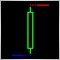# signals from other currency as filter5482

If I would to trade EURUSD, GBPUSD, EURJPY, USDJPY

would it be good if I use this filter?

//+------------------------------------------------------------------+
//| Compare |
//+------------------------------------------------------------------+
int Compare()
{

int S, R;

double Curr_MA, Prev_MA;

if(Symbol() == "EURUSD") S = 1;
if(Symbol() == "GBPUSD") S = 2;
if(Symbol() == "EURJPY") S = 3;
if(Symbol() == "USDJPY") S = 4;

switch(S)
{
case 1 :
Curr_MA = iMA("EURGBP", 0, 5, 0, MODE_EMA, PRICE_CLOSE, 0);
Prev_MA = iMA("EURGBP", 0, 5, 0, MODE_EMA, PRICE_CLOSE, 1);

if(Curr_MA > Prev_MA) R = 1;
if(Curr_MA < Prev_MA) R = 2;

break;

case 2 :
Curr_MA = iMA("EURGBP", 0, 5, 0, MODE_EMA, PRICE_CLOSE, 0);
Prev_MA = iMA("EURGBP", 0, 5, 0, MODE_EMA, PRICE_CLOSE, 1);

if(Curr_MA < Prev_MA) R = 1;
if(Curr_MA > Prev_MA) R = 2;

break;

case 3 :
Curr_MA = iMA("EURUSD", 0, 5, 0, MODE_EMA, PRICE_CLOSE, 0);
Prev_MA = iMA("EURUSD", 0, 5, 0, MODE_EMA, PRICE_CLOSE, 1);

if(Curr_MA > Prev_MA) R = 1;
if(Curr_MA < Prev_MA) R = 2;

break;

case 4 :
Curr_MA = iMA("EURUSD", 0, 5, 0, MODE_EMA, PRICE_CLOSE, 0);
Prev_MA = iMA("EURUSD", 0, 5, 0, MODE_EMA, PRICE_CLOSE, 1);

if(Curr_MA < Prev_MA) R = 1;
if(Curr_MA > Prev_MA) R = 2;

break;
}

return(R);
}1757

Doshur

> If I would to trade EURUSD, GBPUSD, EURJPY, USDJPY - would it be good if I use this filter?

The principle may have some value, more in working out weight of trade (i.e. lot size) rather than entry points IMHO

You should consider that:-

1) Majors drive the crosses rather than the other way round

2) The plan depends on correlation between major pairs which has not been consistent since early '07 IIRC

3) At any one time, one major may be more important than the other in driving a cross, e.g. currently I would be looking at USDJPY to influence a EURJPY position, not EURUSD

4) You have missed one of the strongest correlations, i.e. the tendency of USDCHF to go the opposite way to EURUSD

So...

Much more work needed but you are thinking along useful lines :)

FWIW

-BB-